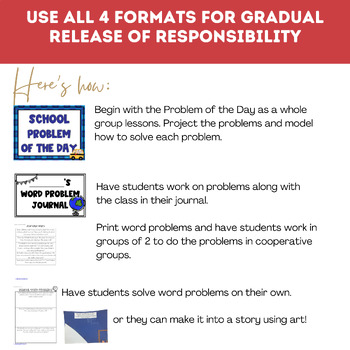# Common Core Math Word Problems: School is Cool!1st - 2nd
Subjects
Standards
Resource Type
Formats Included
• PDF
Pages
18 pages

### Description

These Math Word problems were designed with the Common Core in mind! They were made to use in a first grade classroom, but could also be used in a second grade classroom in the early part of the year. This set includes 5 problems (2 adding, 2 subtracting, 1 three digit) in three formats. In strip form that can be cut and glued into a notebook, a journal that can be run on the copy machine and stapled, or problem of the day pages.

Common Core Standards:

1.OA.1. Use addition and subtraction within 20 to solve word problems involving situations of adding to, taking from, putting together, taking apart, and comparing, with unknowns in all positions, e.g., by using objects, drawings, and equations with a symbol for the unknown number to represent the problem.1

1.OA.2. Solve word problems that call for addition of three whole numbers whose sum is less than or equal to 20, e.g., by using objects, drawings, and equations with a symbol for the unknown number to represent the problem.

2.OA.1. Use addition and subtraction within 100 to solve one- and two-step word problems involving situations of adding to, taking from, putting together, taking apart, and comparing, with unknowns in all positions, e.g., by using drawings and equations with a symbol for the unknown number to represent the problem.

This unit could be used alone or with any of my other Common Core math Word Problem Units. They all have the same format.

Total Pages
18 pages
N/A
Teaching Duration
N/A
Report this Resource to TpT
Reported resources will be reviewed by our team. Report this resource to let us know if this resource violates TpT’s content guidelines.

### Standards

to see state-specific standards (only available in the US).
Use addition and subtraction within 20 to solve word problems involving situations of adding to, taking from, putting together, taking apart, and comparing, with unknowns in all positions, e.g., by using objects, drawings, and equations with a symbol for the unknown number to represent the problem.
Solve word problems that call for addition of three whole numbers whose sum is less than or equal to 20, e.g., by using objects, drawings, and equations with a symbol for the unknown number to represent the problem.